# Scanner Chapter 7 - 11 (Macro Economics) Commerce Notes | EduRev

## Commerce : Scanner Chapter 7 - 11 (Macro Economics) Commerce Notes | EduRev

The document Scanner Chapter 7 - 11 (Macro Economics) Commerce Notes | EduRev is a part of the Commerce Course Economics Class 12.
All you need of Commerce at this link: Commerce

C.B.S.E QUESTION ’S

(Q1) Distinguish between APC and MPC ? The value of which of these two can be greater than one and when ?
(Q2) Explain relationship between APS and MPS ? The value of which of these two can be negative and when ?
(Q3) Distinguish between APC and MPC with the help of numerical examples.

(Q4) Explain any three component of aggregate demand .

(Q5) If national disposable income is Rs.1000 cr and consumption exp. is Rs.750 cr,find APS ?

(Q6) If disposable income is Rs.1200 cr and consumption exp. is Rs.800 cr, find APC ?

(Q7)(a) Income = 500 600 700 800 Calculate MPC and MPS ?
Consump. = 400 480 550 615 Also find APC and APS

(Q7) (b) Income = 1000 1200 1400 1600 Calculate MPC and MPS ?
Consump. = 900 1060 1210 1350

(Q8) Draw on a diagram a straight line saving curve for an economy. From it derive the consumption curve, explaning the method of derivation. Show a point on the consumption curve at which APC is equal to one ?

(Q9) Give meaning of (i) Aggregate demand (ii) Aggregate Supply (iii) Excess Demand

(Q10) Explain the meaning of equilibrium level of national income with the help of diagram ?

(Q11) Explain the equilibrium level of income with the help of saving and investment curves.If saving exceed planned investment what changes will bring about equality between them or what happens when the economy is not in equilibrium and saving exceeds investment ?

(Q12) If in an economy Investment exceeds Saving what will be effect on National income ?

(Q13) Why should planned saving and planned investment be equal at equilibrium level of income?
Explain with diagram ?

(Q14) Why must aggregate demand be equal to aggregate supply at the equilibrium level of income and output ? Explain with the help of a diagram.

(Q15) Explain concept of under-employment equilibrium with the help of diagram.Show on same
diagram the additional investment required to reach full employment equilibrium ?

(Q16) Explain concept of equilibrium level of income with C+ I curve .Can there be unemployment at this level of income??
(Q17) Explain with the help of diagrams that the equilibrium level of income and employment is not necessarily at a full employment level?

(Q18) Explain ‘consumption function’ with the help of a schedule and diagram.

OR
Explain ‘saving function’ with the help of a schedule and diagram.

(6M) (C.B.S.E ‘08)

(Q19) Explain ‘consumption function’ with the help of a schedule. Also record marginal propensity to consume in the schedule.

OR

Explain ‘saving function’ with the help of a schedule. Also record marginal propensity to save in
the schedule.

(6M) ( S.P ‘08)

(Q20) If planned savings are greater than planned investment, what will be its effect on inventories?

(Q21) Give meaning of involuntary unemployment.

(1M) ( S.P ‘08)

(Q22) What is the relationship between marginal propensity to consume and marginal propensity to save?

(1M) ( S.P ‘08)

(Q23) Can an economy be in a state of under employment equilibrium? Explain with the help of
a diagram.

(4M) ( S.P ‘08)

(Q24) Explain the meaning of equilibrium level of income and output with the help of saving and investment curves. If planned expenditure is less than planned output, what changes will take place in the economy ?

(6M) ( S.P ‘08)

(Q25) Define ‘aggregate supply’.

(1M) ( S.P ‘08)

(Q26) Given below is the consumption function in an economy : C = 100 + 0.5Y
With the help of a numerical example show that in this economy as income increases APC will
decrease.

(6M) ( S.P ‘08)

C.B.S.E ‘09

(Q1) Give meaning of aggregate supply.

(1M)

(Q2) Give the meaning of excess demand in an economy.

(1M)

(Q3) Define involuntary unemployment.

(1M)

(Q4) From the following information about an economy, calculate
(i) its equilibrium level of national income, and
(ii) consumption expnditure at equilibrium level of national income.
Consumption function : C = 500 + 0.9 Y and I = 1000 cr

(Q5) In an economy S = - 50 + 0.5 Y is the saving function
(where S = saving and Y = national income) and investment expenditure is 7000. Calculate :
(i) Equilibrium level of national income.
(ii) Consumption expenditure at equilibrium level of national income.
OR
From the following information about an economy, calculate
(i) its equilibrium level of national income, and
(ii) savings at equilibrium level of national income.
Consumption function : C = 200 + 0.9 Y and Investment expenditure : I = 3000
(where C = consumption expenditure and Y = national income)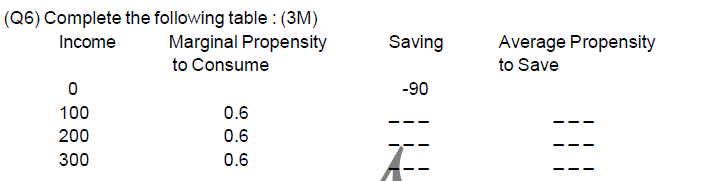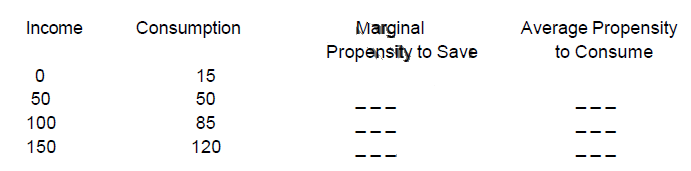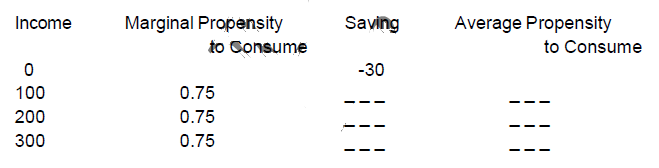(Q7) What changes will take place to bring an economy in equilibrium if
(a) planned savings are greater than planned investment and
(a) planned savings are less than planned investment

SAMPLE PAPER + C.B.S.E 2010

(Q1) What is ex-ante aggregate demand ?

(1M)

(Q2) Give the meaning of marginal propensity to save.

(1M)

(Q3) When is there the equilibrium level of national income?

(1M)

(Q4) Give the meaning of involuntary unemployment.

(1M)

(Q5) What is the relationship between marginal propensity to save and marginal propensity to consume?

(1 M)

(Q6) Can an economy be in equilibrium when there is unemployment in the economy. Explain.

(3 M)

(Q7) Explain the meaning of equilibrium level of income and output using savings and investment approach. Use a diagram.

(4 M)

(Q8) Giving reasons, state whether the following statements are true or false :

(4M)

(a) Value of average propensity to save can never be less than zero.
(b) Sum of average propensity to consume and marginal propensity to consume is
always equal to one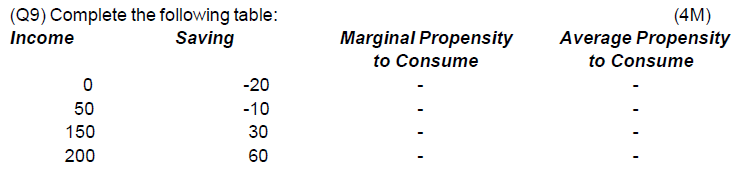(Q10) Draw a straight line consumption curve. From it derive a savings curve explaining the
process. Show on this diagram:

(6 M)

(a) the level of income at which Average Propensity to Consume is equal to one.
(b) a level of income at which Average Propensity to Save is negative.

(Q11) Given below is the consumption function in an economy:

(6 M)

C = 100 + 0.5Y
With the help of a numerical example show that in this economy as income increases APC will decrease.

(Q12) Giving reasons, state whether the following statements are true or false :

(4M)

(a) Value of average propensity to save can never be greater than one
(b) Value of MPS can never be negative

(Ans :: T,T)

C.B.S.E PAPER 2011 & 2012

(Q 1) Explain the distinction between voluntary and involuntary unemployment.What is the
significance of these distinction

(3 marks)

(Q2) Find National Income from the following :

(3 marks)

Autonomous Consumption = Rs.100
Marginal propensity to consume = 0.60
Investment = Rs. 200 Ans. 750 crore.

(Q3) Find Investment from the following :

(3 marks)

National Income = Rs. 600
Autonomous Consumption = Rs. 150
Marginal propensity to consume = 0.70 Ans. 30 crore.

(Q4) Find Consumption Expenditure from the following :

(3 marks)

National Income = Rs. 5,000
Autonomous Consumption = Rs. 1,000
Marginal propensity to consume = 0.80 Ans. 5,000

(Q5) Outline the steps taken in deriving Consumption Curve from the Saving Curve. Use diagram.

OR
Distinguish between propensity to consume and propensity to save, with the help of numerical examples.

(3 marks)

(Q6) Given that national income is Rs. 50 crore and Saving is 5 crore, find out average
propensity to consume . When income rises to Rs. 60 crore and saving to 9 crore , what will be
the average propensity to consume and the marginal propensity to save ?

(Ans. 0.90; 0.85; 0.40)

(Q7) Given that national income is Rs. 90 crore and consumption expenditure Rs. 81 crore, find
out average propensity to save. When income rises to Rs. 100 crore and consumption
expenditure to Rs. 88 crore, what will be the marginal propensity to consume and the marginal
propensity to consume ?

(Ans. 0.10; 0.70; 0.30)

C.B.S.E PAPER 2013

(Q1) From the data given below about an economy, calculate (a) investment expenditure and
(b) consumption expenditure. (6 M)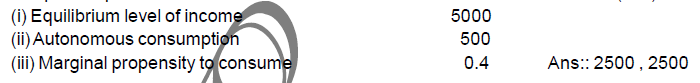(Q2) Explain the meaning of under-employment equilibrium. Explain two measures by which
full-employment equilibrium can be reached.

(Q3) Explain all the changes that will take place in an economy when aggregate demand is not
equal to aggregate supply.

(6 M)

(Q4) In an economy C = 200 + 0.75Y is the consumption function where C is consumption
expenditure and Y is national income. Investment expenditure is 4000. Calculate equilibrium
level of income and consumption expenditure.

(6 M)

Ans:: 16800 ,12800

(Q5) From the following data about an economy, calculate (a) equilibrium level of national
income and (b) total consumption expenditure at equilibrium level of national income.
(i) C = 200 + 0.5Y is the consumption function where C is consumption expenditure and
Y is national income.
(ii) Investment expenditure is 1500.

(6 M)

Ans:: 3400 ,1900

(Q6) In an economy S = - 100 + 0.6Y is the saving function where S is saving and Y is national
income. Investment expenditure is 1100. Calculate equilibrium level of income and
consumption expenditure.

Ans :: 2000 ,900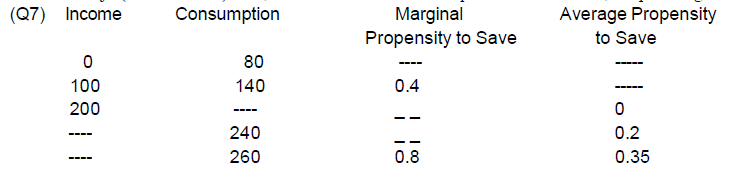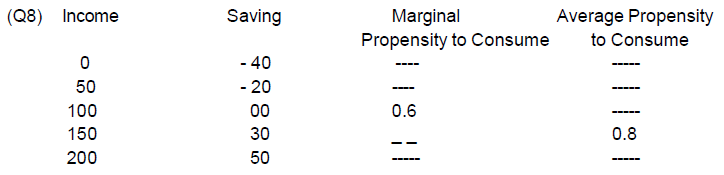C. B. S. E PAPER 2014

(Q1) What is full employment ?

(Q2) Outline the steps required to be taken in deriving the Consumption Curve from the given Saving Curve. Use diagram.

(6 M)

(Q3) When is an economy in equilibrium ? Explain with the help of Saving and Investment functions. Also explain the changes that take place in an economy when the economy is not in equilibrium. Use diagram.

(Q4) Explain national income determination through the two alternative approaches ? Use diagram

(Q5) Calculate Marginal Propensity to Consume from the following data about an economy which is in equilibrium :
National Income = 2000
Autonomous consumption expenditure = 200
Investment expenditure = 100 Ans :: 0.85

(Q5) Calculate Marginal Propensity to Consume from the following data about an economy
which is in equilibrium :
National Income = 1500
Autonomous consumption expenditure = 300
Investment expenditure = 300 Ans :: 0.6

(Q6) Calculate investment expenditure from the following data about an economy which is equilibrium :
National income = 1000
Marginal propensity to save = 0.20
Autonomous consumption expenditure = 100 (4 marks)

Ans :: 100

(Q7) Calculate investment expenditure from the following data about an economy which is equilibrium :
National income = 1000
Marginal propensity to save = 0.25
Autonomous consumption expenditure = 200

Ans :: 50

(Q8) Calculate Autonomous Consumption Expenditure from the following data about an economy
which is in equilibrium :
National income = 1200
Marginal propensity to save = 0.20
Investment expenditure = 100

Ans :: 140

(Q9) Calculate Autonomous Consumption Expenditure from the following data about an economy
which is in equilibrium :
National income = 500
Marginal propensity to save = 0.30
Investment expenditure = 100

Ans :: 50

SAMPLE PAPER
(Q1) What is involuntary unemployment ?

(1 mark)

(Q2) Draw a straight line consumption curve. From it derive a saving curve. Explain the process
of derivation. Show in this diagram :

(6 marks)

(a) The point at which the Average propensity to Consume is equal to one.
(b) Any point at which the Average Propensity to Save in negative

(Q3) Using the ‘saving and investment’ approach explain how is the equilibrium level of national income determined ? Also explain what will happen if the equilibrium condition is not fulfilled.

(Q4) From the following information, calculate equilibrium level of income :

(3 marks)

(i) Investment Rs. 80
(ii) Marginal propensity to consume 0.8
(iii) Consumption expenditure at zero income Rs. 40

(Q5) In an economy, consumption function is C = 400 + 0.7Y where, C = Consumption and Y is
National Income and Investment Expenditure is 2000. Calculate equilibrium level of national
income and consumption expenditure.

(6 marks)

CBSE 2015 & SAMPLE PAPER

(Q1) What is ‘aggregate demand’ in macroeconomics ?

(1 M)

Ans :: Value of final products the buyers are planning to buy during a given period at a given level of income.

(Q2) Explain how the economy achieves equilibrium level of income using Savings-Investment (S-I) approach.

(3M)

Ans :: As per the S - I approach equilibrium if achieved where ex-ante Savings are equal to ex-ante investments. Savings and investments indicate leakages and injections respectively, thus at equilibrium the leakages and injections are equal to each other.

(Q3) Give the meaning of (a) Autonomous Consumption (b) Full employment

(Q4) Which of these cannot have a negative value ?Give reasons .
(a) APS (b) MPS

(Q5) An economy is in equilibrium. Calculate the Investment Expenditure from the following :
National Income = 800 , Marginal Propensity to Save = 0.3 , Autonomous Consumption = 100

Ans :: Y = C + MPC(Y) + I (11/2 )
800 = 100 + (1 – 0.3)800 + I (2 M)
I = 800 – 100 – 560 = 140 (1/2 )
(No marks if only the final answer is given)

(Q6) An economy is in equilibrium. Calculate the National Income from the following :

(4 M)

Autonomous Consumption = 120 , Marginal Propensity to Save = 0.2 ,
Investment Expenditure = 150

Ans :: 1350

(Q7) An economy is in equilibrium. Calculate the Marginal Propensity to Save from the
following: National Income = 1000 (4 M)
Autonomous Consumption = 100
Investment = 120

Ans :: 0.22

(Q8) If in an economy Saving function is given by S = (-) 50 + 0.2 Y and Y = Rs.2000 crores; consumption expenditure for the economy would be Rs.1,650 crores and the autonomous investment is Rs.50 crores and the marginal propensity to consume is 0.8. True or False? Justify your answer with proper calculations.

OR
“Economists are generally concerned about the rising Marginal Propensity to Save (MPS) in an
economy”. Explain why?

(3M)

Ans :: Yes all the given values are correct

OR

Ans :: Since the sum of MPC and MPS is unity any increase in Marginal Propensity to Save (MPS) would directly lead to decrease in Marginal Propensity to Consume (MPC). This means that may lead to lesser proportion of the additional income going to consumption which is a vital factor of Aggregate Demand/Expenditure. This may further lead to fall in equilibrium level of income in the economy.

(Q9) In an economy the autonomous investment is 100 and the consumption is C=80+0.4Y. Is the economy in equilibrium at an income level 400? Justify your answer.

(3M)

OR
In an economy the autonomous investment is 60 and the marginal propensity to consume is 0.8.
If the equilibrium level of income is 400, then the autonomous consumption is 30. True or False?

(1M)

Ans :: At equilibrium AD = Y AD = C+I = Y

80+0.4Y+100 = Y
0.6Y = 180
Y = 300

(1M)

Since the given income of 400 is greater than equilibrium level of income, the economy is not at equilibrium. It is at a situation where aggregate demand is greater than the aggregate output produced in the economy.

(1M)

OR
The given value of autonomous consumption is incorrect. The correct value is 20.

(Q10) Derive a straight line saving curve using the following consumption function:

(6M)

C = 20 + 0.6Y.
Presuming the income levels to be Rs.100, Rs. 200 and Rs. 300 crores. Also calculate that level of income where consumption is equal to income.

(Q11) In an economy planned saving is greater than planned investment. Explain how the economy
achieves equilibrium level of national income.

(3M)

Ans :: Suppose planned saving is higher than planned investment. It means that households are not consuming as much as the firms had anticipated. In other words, planned output is greater than planned demand.
As a result, producers see a rise in their inventory level, beyond the planned level. To bring back inventory to the planned level, producers cut down production. This reduces aggregate output. The process continues till aggregate demand equals the output produced in the economy i.e. planned investment becomes equal to planned saving.

CBSE 2016

(Q1) What is aggregate demand ? State its components.

(3M)

(Q2) Distinguish between marginal propensity to consume and average propensity to consume. Give a numerical example

(Q3) Given saving curve, derive consumption curve and state the steps used . Use diagram.

(Q4) Given consumption curve, derive saving curve and state the steps taken in the process of derivation. Use diagram.

(6M)

(Q5) Derive the two alternative conditions of expressing national income equilibrium . Show these on a single diagram .

(Q6) An economy is in equilibrium. Find investment expenditure :
National income = 1200
Autonomous consumption expenditure = 150
Marginal Propensity to consume = 0.8

Ans :: 90

(Q7) An economy is in equilibrium. Find investment expenditure :
National Income = 1000 , Autonomous Consumption = 100 , MPC = 0.8

Ans :: 100
(Q8) An economy is in equilibrium. Calculate Marginal Propensity to Consume :

(3M)

National income = 1000
Autonomous consumption expenditure = 200
Investment expenditure = 100

Ans :: 0.7

(Q9) Explain the meaning of under -employment equilibrium ?

(c)

(Q10) In an economy ,planned spending is greater than planned output .Explain all the changes
that will take place in the economy ?

(c) , 0r

In an economy ,planned spending is greater than planned output .Explain all the changes that will take place in the economy ?

(Q11) Explain all the changes that will take place in the economy when aggregate demand and aggregate supply are not equal

(c)

(Q12) S = - 10 + 0.2 Y is the saving function in an economy . Investment exp. is 5,000. Calculate
equilibrium level of income

Ans 25,500

EXTRA QUESTION’S

(Q1) Does equilibrium beyond full employment implies a higher level of output compared to full employment equilibrium ?

(Q2) What is meant by AD and AS? Will there be Full employment when these two are equal ?

(Q3) Differenciates between F.E.L and U.E.L ?

(Q4) What is aggregate effective demand ? ( Demand which is actually spent)

(Q5) Give meaning of AD ? How does budgetary Deficit effect it?

(Q6) Name the principle component of aggregate demand

(Q7) What determines the size of investment ?

(Q8) What determines the level of household consumption demand in an economy ?

(Q9) How is AS function in the classical framework different from AS function in the Keynesian
framework ?
(Q10) Define Consumption function, saving Function and Investment demand function ?

(Q11) Differentiate between full employment and underemployment ?

(Q12) What are the important elements important in understanding investment ?

(Q13) What happens to national income when AD falls short of AS ?

(Q14) What will be the behaviour of level of income , when ex-ante saving are lesser than
ex-ante investment ?

(Q15) When does autonomous investment becomes essential ?

(Q16) Exlplain the component of equation C = c + by

(Q17) How is AS measured or What are components of AS ?

(Q18) Using the equation C = 20 + .9Y. Construct a schedule for consumption where income
200, 250, 300, 350, 400. Also derive saving function.

(Q19) Do you think increase in MPS should be beneficial to the growth of GDP in INDIA ?

Ans :: if it is banked and banks invests it . In india banking habits are poor and saving remains
as idle cash and causes deficiency of AD and decreases GDP

(Q20) “The export fall 15 % to 1718.07cr in first 6 months”. How will this affect AD in the economy?

(Q21) What are “desired stock” with the producer ?

Ans :: It is that level of stock where AS = AD and the producers are in the state of equilibrium

(Q22) The producer suffer losses when actual stocks are less than the desired stocks.

( Ans True ( loss of unfilled demand )

(Q23) In the Keynesian model , short period equilibrium is discussed with reference to constant
price level ?

( Ans : true )

(Q24) Equilibrium beyond full employment is a better situation than equilibrium at full mployment.
Defend or Refute ?

(Q25) Exemption limit for the payment of income tax has been raised from 2 lakh to 2.5 lakh for
the financial year 2015 -16 . How would it affect aggregate consumption if MPC is assumed to
remain constant ?

Offer running on EduRev: Apply code STAYHOME200 to get INR 200 off on our premium plan EduRev Infinity!

## Economics Class 12

224 videos|237 docs|54 tests

,

,

,

,

,

,

,

,

,

,

,

,

,

,

,

,

,

,

,

,

,

;